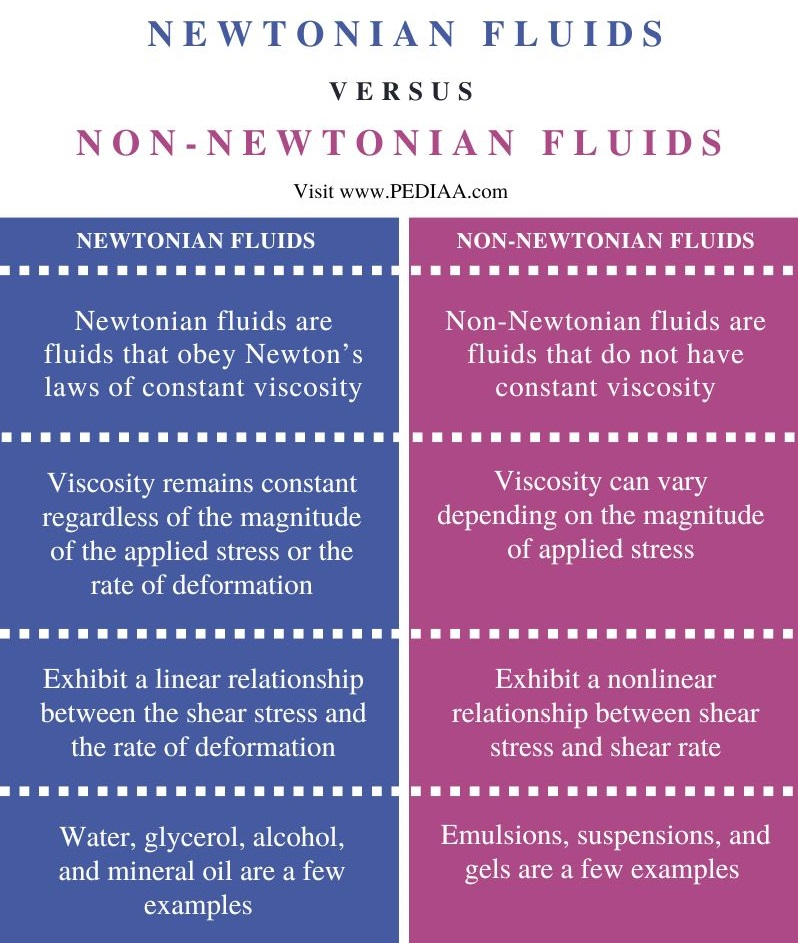# What is the Difference Between Newtonian and Non-Newtonian Fluids

The main difference between Newtonian and non-Newtonian fluids is that Newtonian fluids are fluids that obey Newton’s laws of constant viscosity, whereas Non-Newtonian fluids are fluids that do not have constant viscosity.

Fluid is a liquid, gas, or other material that continually deforms under an applied shear stress or external force. Thus, fluids have the ability to flow and take the shape of a container they occupy. Also, some common properties of fluids include density, viscosity, and compressibility. Based on their properties, fluids can be classified as Newtonian fluids and non-Newtonian fluids. Understanding the properties and behavior of these fluids is essential in many fields, including engineering, .physics, chemistry, and biology.

### Key Areas Covered

1. What are Newtonian Fluids
– Definition, Viscosity, Features
2. What are Non-Newtonian Fluids
– Definition, Viscosity, Features
3. Difference Between Newtonian and Non-Newtonian Fluids
– Comparison of Key Differences

### Key Terms

Newtonian Fluids, Non-Newtonian Fluids## What are Newtonian Fluids

Newtonian fluids are fluids that follow Newton’s laws of viscosity. This law indicates that the shear stress of a fluid is proportional to the rate of its deformation, with the constant of proportionality being the viscosity of the fluid. This means that the viscosity of a Newtonian fluid remains constant regardless of the magnitude of the applied stress or the rate of deformation. Water, glycerol, alcohol, and mineral oil are a few examples of Newtonian fluids.Newtonian fluids have the ability to flow freely when subjected to applied stress. This is because the viscosity of Newton’s fluid does not change with the magnitude of the applied stress. Hence, these fluids can be easily pumped or transported through pipes and channels without significant changes in their flow properties. They also exhibit lamina flow behavior at low flow rates and high viscosities. Lamina flow occurs when a fluid flows in layers, with each layer moving in a parallel direction to the adjacent layer.

Newtonian fluids also have some limitations. These fluids may not exhibit the flow properties of real-world fluids such as blood.

## What are Non-Newtonian Fluids

Non-Newtonian fluids are fluids that do not follow Newton’s law of viscosity. Depending on the magnitude of applied stress, the viscosity of non-Newtonian fluids can vary. Emulsions, suspensions, and gels are examples of non-Newtonian fluids.Non-Newtonian fluids exhibit shear thickening behavior or shear thinning behavior. Shear thickening behavior occurs when the viscosity of a fluid increases with the magnitude of the applied stress, while shear thinning behavior occurs when the viscosity of a fluid decreases with the magnitude of the applied stress. These liquids also show complex flow patterns such as vortex formation, shear banding, and flow instabilities. These flow patterns can significantly impact the transport and mixing properties of non-Newtonian fluids. They also exhibit time-dependent behavior, such as viscoelasticity. This behavior is commonly observed in polymer solutions and gels.

## Difference Between Newtonian and Non-Newtonian Fluids

### Definition

Newtonian fluids are fluids that obey Newton’s laws of constant viscosity, whereas Non-Newtonian fluids are fluids that do not have constant viscosity.

### Viscosity

In a Newtonian fluid, the viscosity remains constant regardless of the magnitude of the applied stress or the rate of deformation, while the viscosity of non-Newtonian fluids can vary depending on the magnitude of applied stress.

### Flow Behaviour

Newtonian fluids exhibit a linear relationship between the shear stress and the rate of deformation, while non-Newtonian fluids exhibit a nonlinear relationship between shear stress and shear rate.

### Examples

Water, glycerol, alcohol, and mineral oil are a few examples of Newtonian fluids, while emulsions, suspensions, and gels are examples of non-Newtonian fluids.

### Conclusion

Fluids are liquids, gasses, or other materials that continually deform under an applied shear stress or external force. Fluids can be classified as Newtonian fluids and non-Newtonian fluids. The main difference between Newtonian and non-Newtonian fluids is that Newtonian fluids obey Newton’s laws of constant viscosity, whereas Non-Newtonian fluids do not have constant viscosity.

##### Reference:

1. “Newtonian Fluid – Definition, Examples, Industrial Applications.” Byju’s Exam Prep.
2. “Non-Newtonian fluids.” Science Learning Hub.

##### Image Courtesy:

1. “Water Liquid Research Lab” (CC0) via Pxhere
2. “Newtonian vs Non-Newtonian 1” By Joseasorrentino – Own work (CC BY-SA 4.0) via Commons Wikimedia